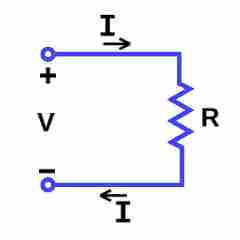Science >> Physics for Kids

# Physics for Kids

## Electric Current

Current is the flow of an electric charge. It is an important quantity in electronic circuits. Current flows through a circuit when a voltage is placed across two points of a conductor.

Flow of Electrons

In an electronic circuit, the current is the flow of electrons. However, generally current is shown in the direction of the positive charges. This is actually in the opposite direction of the movement of the electrons in the circuit.

How is current measured?

The standard unit of measurement for current is the ampere. It is sometimes abbreviated as A or amps. The symbol used for current is the letter "i".

Current is measured as the flow of electric charge over time through a given point in an electric circuit. One ampere is equal to 1 coulomb over 1 second. A coulomb is a standard unit of electric charge.

Calculating Current

Current can be calculated using Ohm's Law. It can also be used to figure out the resistance of a circuit if the voltage is also known or the voltage of a circuit if the resistance is known.

I = V/R
where I = current, V = voltage, and R = resistanceCurrent is also used to calculate power using the following equation:

P = I * V
where P = power, I = current, and V = voltage.

AC versus DC

There are two main types of current used in most electronic circuits today. They are alternating current (AC) and direct current (DC).
• Direct Current (DC) - Direct current is the constant flow of electric charge in one direction. Batteries generate direct current to power handheld items. Most electronics use direct current for internal power often converting alternating current (AC) to direct current (DC) using a transformer.
• Alternating Current (AC) - Alternating current is current where the flow of electric charge is constantly changing directions. Alternating current is mostly used today to transmit power on power lines. In the United States the frequency at which the current alternates is 60 Hertz. Some other countries use 50 Hertz as the standard frequency.
Electromagnetism

Current also plays an important role in electromagnetism. Ampere's law describes how a magnetic field is generated by an electric current. This technology is used in electric motors.

• The direction of the current flow is often shown with an arrow. In most electronic circuits the current is shown as flowing towards ground.
• The current in a circuit is measured using a tool called an ammeter.
• The flowing of electric current through a wire can sometimes be thought of like the flowing of water through a pipe.
• The electrical conductivity of a material is the measurement of the ability of the material to allow for the flow of electrical current.
Activities

More Electricity Subjects

Science >> Physics for Kids